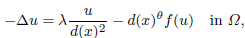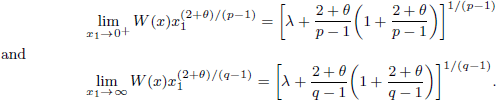Home

Asymptotic behaviour and symmetry of positive solutions to nonlinear elliptic equations in a half-space

Extract

We consider the following equation:where d(x) = d(x, ∂Ω), θ > –2 and Ω is a half-space. The existence and non-existence of several kinds of positive solutions to this equation when, f(u) = up (p > 1) and Ω is a bounded smooth domain were studied by Bandle, Moroz and Reichel in 2008. Here, we study exact the behaviour of positive solutions to this equation as d(x) → 0+ and d(x) → ∞, respectively, and the symmetry of positive solutions when, Ω is a half-space and f(u) is a more general nonlinearity term than up . Under suitable conditions for f, we show that the equation has a unique positive solution W, which is a function of x 1 only, and W satisfiesMetrics

Full text viewsFull text views reflects the number of PDF downloads, PDFs sent to Google Drive, Dropbox and Kindle and HTML full text views.

Total number of HTML views: 0
Total number of PDF views: 0 *Loading metrics...

Abstract viewsAbstract views reflect the number of visits to the article landing page.

Total abstract views: 0 *Loading metrics...

* Views captured on Cambridge Core between <date>. This data will be updated every 24 hours.

Usage data cannot currently be displayed